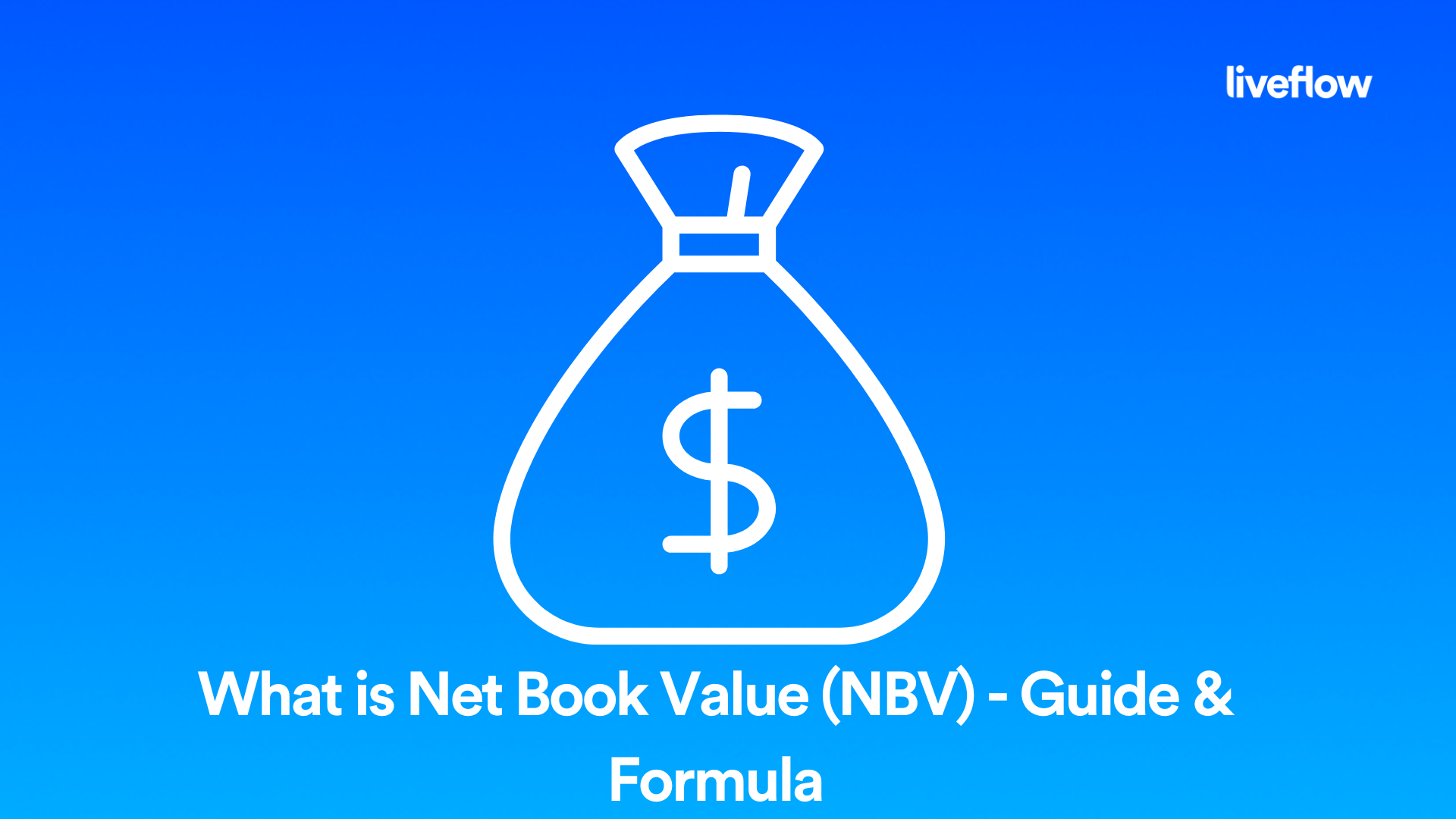# What is Net Book Value (NBV) - Guide & Formula

July 27, 2022Net book value (NBV) is an important concept in accounting, and it's something that all business owners should understand. In this blog post, we will introduce you to NBV and explain what it is and how to calculate net book value. We will also discuss how our accounting product, LiveFlow, can help you calculate net book value for your business.

## How do you calculate net book value?

The net book value of an asset is the difference between the historical cost of the asset and any accumulated depreciation.

For example, if you purchased a car for \$20,000 and it has depreciated by \$15,000 over its useful life, then the net book value of the car would be \$20,000 - \$15,000 = \$5000.

### What is the net book value of a business?

The net book value of a business is the sum of all its assets, minus any liabilities and intangible assets. So, if your business has assets worth \$100,000 and liabilities of \$50,000, then the net book value of the business would be \$100,000 - \$50,000 = \$50,000.

### What is net book value in accounting?

Net book value in accounting is the historical cost of an asset minus any accumulated depreciation. It's a way to measure the value of an asset for balance sheet purposes.

## What is net book value and how is it calculated?

The net book value of an asset is the historical cost of the asset minus any accumulated depreciation. To calculate net book value, you'll need to know the original purchase price of the asset and how much it has depreciated over time.

### What is the net book value formula?

The net book value formula is:

Net book value = Historical cost - Accumulated depreciation

For example, let's say you purchased a piece of equipment for \$100,000. Over the course of five years, it has depreciated by \$50,000. This means its net book value would be \$100,000 - \$50,000, or \$50,000.

## What is the difference between book value and net book value?

The book value of an asset is its historical cost, net of any impairments. The net book value is the book value minus any accumulated depreciation.

So, if you have an asset that was purchased for \$100,000 and it has depreciated by \$50,000 over five years, the net book value would be \$50,000. The book value would be \$100,000.

### Why is net book value important?

Net book value is important because it provides a snapshot of an asset's value at a specific point in time. This information can be useful for making decisions about whether to keep or sell an asset. It can also be used to calculate the amount of depreciation expense to record on an income statement.

Net book value is just one factor to consider when making decisions about your assets. However, it can be a helpful tool in understanding the current value of your equipment and other property.

If you're looking for an easy way to calculate net book value, check out LiveFlow's accounting software. Our net book value calculator will help you determine the value of your assets quickly and easily.

## What is LiveFlow?

LiveFlow is an accounting software that helps business owners manage their finances. Our software templates include a net book value calculator to help users track the value of their assets. We also offer a variety of other features, such as invoicing, tax preparation, and financial reporting.